Search by Topic

Resources tagged with Graphs similar to Curve Fitter:

Filter by: Content type:
Age range:
Challenge level:

There are 43 results

Broad Topics > Functions and Graphs > GraphsCurve Fitter

Age 16 to 18 Challenge Level:

Can you fit a cubic equation to this graph?Curve Match

Age 16 to 18 Challenge Level:

Which curve is which, and how would you plan a route to pass between them?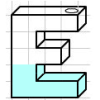Mathsjam Jars

Age 14 to 16 Challenge Level:

Imagine different shaped vessels being filled. Can you work out what the graphs of the water level should look like?Parabolic Patterns

Age 14 to 18 Challenge Level:

The illustration shows the graphs of fifteen functions. Two of them have equations y=x^2 and y=-(x-4)^2. Find the equations of all the other graphs.Exploring Cubic Functions

Age 14 to 18 Challenge Level:

Quadratic graphs are very familiar, but what patterns can you explore with cubics?More Parabolic Patterns

Age 14 to 18 Challenge Level:

The illustration shows the graphs of twelve functions. Three of them have equations y=x^2, x=y^2 and x=-y^2+2. Find the equations of all the other graphs.Parabolas Again

Age 14 to 18 Challenge Level:

Here is a pattern composed of the graphs of 14 parabolas. Can you find their equations?Guess the Function

Age 16 to 18 Challenge Level:

This task depends on learners sharing reasoning, listening to opinions, reflecting and pulling ideas together.Four on the Road

Age 14 to 16 Challenge Level:

Four vehicles travel along a road one afternoon. Can you make sense of the graphs showing their motion?Age 14 to 16 Challenge Level:

Four vehicles travelled on a road. What can you deduce from the times that they met?Cubic Spin

Age 16 to 18 Challenge Level:

Prove that the graph of f(x) = x^3 - 6x^2 +9x +1 has rotational symmetry. Do graphs of all cubics have rotational symmetry?Motion Sensor

Age 14 to 16 Challenge Level:

Looking at the graph - when was the person moving fastest? Slowest?Which Is Bigger?

Age 14 to 16 Challenge Level:

Which is bigger, n+10 or 2n+3? Can you find a good method of answering similar questions?Ellipses

Age 14 to 18 Challenge Level:

Here is a pattern for you to experiment with using graph drawing software. Find the equations of the graphs in the pattern.Parabella

Age 16 to 18 Challenge Level:

This is a beautiful result involving a parabola and parallels.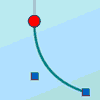Graphical Interpretation

Age 14 to 16 Challenge Level:

This set of resources for teachers offers interactive environments to support work on graphical interpretation at Key Stage 4.Small Steps

Age 16 to 18 Challenge Level:

Two problems about infinite processes where smaller and smaller steps are taken and you have to discover what happens in the limit.Real(ly) Numbers

Age 16 to 18 Challenge Level:

If x, y and z are real numbers such that: x + y + z = 5 and xy + yz + zx = 3. What is the largest value that any of the numbers can have?Which Is Cheaper?

Age 14 to 16 Challenge Level:

When I park my car in Mathstown, there are two car parks to choose from. Can you help me to decide which one to use?Matchless

Age 14 to 16 Challenge Level:

There is a particular value of x, and a value of y to go with it, which make all five expressions equal in value, can you find that x, y pair ?Bio Graphs

Age 14 to 16 Challenge Level:

What biological growth processes can you fit to these graphs?What's That Graph?

Age 14 to 16 Challenge Level:

Can you work out which processes are represented by the graphs?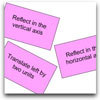Surprising Transformations

Age 14 to 16 Challenge Level:

I took the graph y=4x+7 and performed four transformations. Can you find the order in which I could have carried out the transformations?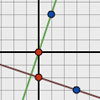Perpendicular Lines

Age 14 to 16 Challenge Level:

Position the lines so that they are perpendicular to each other. What can you say about the equations of perpendicular lines?Gosh Cosh

Age 16 to 18 Challenge Level:

Explore the hyperbolic functions sinh and cosh using what you know about the exponential function.After Thought

Age 16 to 18 Challenge Level:

Which is larger cos(sin x) or sin(cos x) ? Does this depend on x ?Climbing

Age 16 to 18 Challenge Level:

Sketch the graphs of y = sin x and y = tan x and some straight lines. Prove some inequalities.Power Up

Age 16 to 18 Challenge Level:

Show without recourse to any calculating aid that 7^{1/2} + 7^{1/3} + 7^{1/4} < 7 and 4^{1/2} + 4^{1/3} + 4^{1/4} > 4 . Sketch the graph of f(x) = x^{1/2} + x^{1/3} + x^{1/4} -xMaths Filler 2

Age 14 to 16 Challenge Level:

Can you draw the height-time chart as this complicated vessel fills with water?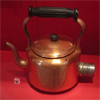Electric Kettle

Age 14 to 16 Challenge Level:

Explore the relationship between resistance and temperature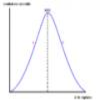Graphic Biology

Age 16 to 18 Challenge Level:

Several graphs of the sort occurring commonly in biology are given. How many processes can you map to each graph?Immersion

Age 14 to 16 Challenge Level:

Various solids are lowered into a beaker of water. How does the water level rise in each case?Golden Construction

Age 16 to 18 Challenge Level:

Draw a square and an arc of a circle and construct the Golden rectangle. Find the value of the Golden Ratio.Exponential Trend

Age 16 to 18 Challenge Level:

Find all the turning points of y=x^{1/x} for x>0 and decide whether each is a maximum or minimum. Give a sketch of the graph.Equation Matcher

Age 16 to 18 Challenge Level:

Can you match these equations to these graphs?Whose Line Graph Is it Anyway?

Age 16 to 18 Challenge Level:

Which line graph, equations and physical processes go together?How Many Solutions?

Age 16 to 18 Challenge Level:

Find all the solutions to the this equation.Without Calculus

Age 16 to 18 Challenge Level:

Given that u>0 and v>0 find the smallest possible value of 1/u + 1/v given that u + v = 5 by different methods.Three Ways

Age 16 to 18 Challenge Level:

If x + y = -1 find the largest value of xy by coordinate geometry, by calculus and by algebra.Interpolating Polynomials

Age 16 to 18 Challenge Level:

Given a set of points (x,y) with distinct x values, find a polynomial that goes through all of them, then prove some results about the existence and uniqueness of these polynomials.Lap Times

Age 14 to 16 Challenge Level:

Can you find the lap times of the two cyclists travelling at constant speeds?Real-life Equations

Age 16 to 18 Challenge Level:

Here are several equations from real life. Can you work out which measurements are possible from each equation?Bus Stop

Age 14 to 16 Challenge Level:

Two buses leave at the same time from two towns Shipton and Veston on the same long road, travelling towards each other. At each mile along the road are milestones. The buses' speeds are constant. . . .